Next: Theorem 1 Up: Declarative Formalization of Strategies Previous: Introduction

# Blocks World Example

In this section and the next, we show how the ideas outlined above can be applied to formalize and reason about heuristics for moving blocks in order to solve planning problems in the blocks world. The strategies formalized in the paper describe different algorithms for solving planning problems in the elementary blocks world domain [Gupta & Nau1991]. First, we summarize a very elegant and simple formalization of STRIPS in the situation calculus, and its application to reasoning about action in the blocks world, described in [McCarthy1985]. Then, we propose a nested abnormality theory (NAT) [Lifschitz1995] that solves the problem of projecting the strategy described by axiomsto. In section 3, this theory is generalized into a class of NAT's which apply circumscription in a particular way that is useful for studying the projections of declarative formalizations of strategies of the sort described in this paper.

In [McCarthy1985], John McCarthy proposes a very simple formalization of STRIPS [Fikes & Nilsson1971] in the situation calculus. The formalization is as follows. STRIPS is a planning system that uses a database of logical formulas to represent information about a state. Each action has a precondition, an add list, and a delete list. When an action is considered, it is first determined whether its precondition is satisfied. If the precondition is met, then the sentences on the delete list are deleted from the database, and the sentences on the add list are added to it.

There are variables of the following sorts: for situations (s,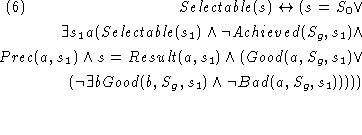, ...), for actions (a,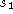, ...), and for propositional fluents (p,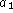, ... ). Associated with each situation is a database of propositions, and this gives us the wff DB(p,s) asserting that p is in the database associated with s. The function Result maps a situation s and an action a into the situation that results when action a is performed in situation s.

STRIPS is characterized by three predicates: (1) Prec(a,s) is true provided action a can be performed in situation s; (2) Delete(p,a,s) is true if proposition p is to be deleted when action a is performed in situation s; (3) Add(p,a,s) is true if proposition p is to be added when action a is performed in situation s. STRIPS has the single axiom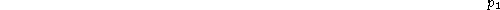In [McCarthy1985], an example of how to use this formalization of STRIPS to reason about action in the blocks world is given. The example is as follows (we have modified the initial conditions, and added a uniqueness of names axiom). The variables x, y and z range over blocks. The constant blocks used in the example are A, B, C, D, E, F, and T (for Table). The one kind of propositional fluent is On(x,y) describing the fact that block x is on block y. The one kind of action is Move(x,y) denoting the act of moving block x on top of block y. We assume uniqueness of names for every function symbol, and every pair of distinct function symbols. The initial situation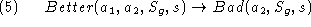is described by axiom. The precondition, delete and add lists of Move(x,y) are characterized by axioms,and, respectively.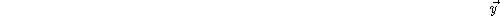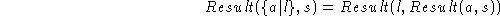In order to interpret action selection rules, such as axiomsto, in terms of the theory of action described above, we need to establish a connection between what holds at a situation and what is in the database associated with that situation.

The databases in the formalization of the blocks world presented above contain only propositional fluents of the form On(x,y) for x, y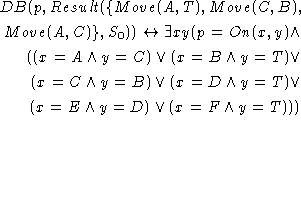{A,B,C,D,E,F,T}. These propositional fluents are called frame fluents [McCarthy & Hayes1969] [Lifschitz1990], since any configuration of the blocks A,B,C,D,E, and F can be described by combinations of their values.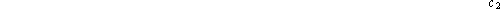The following axiom states that a frame fluent holds at a particular situation if and only if it is in the database associated with that situation.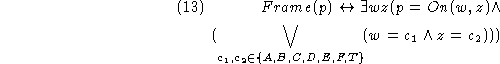The expression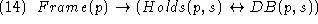means that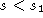can be reached [Reiter1993] from s by executing a nonempty sequence of actions (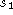is an abbreviation for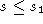). We include domain closure axioms for blocks, actions and situations. Axiomrestricts the domain of situations to those that can be reached from the initial situationor from the goal situation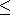. Finally, we introduce an axiom of induction for situations, which allows us to prove that a property holds for all the situations that can be reached from a given situation.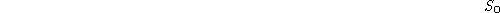In addition to frame fluents, we use a number of derived fluents, such as clear, final, above, tower-deadlock, terminal, and achieved, which are partiallydefined in terms of the frame fluents.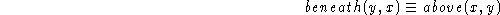Axiomdescribes the configuration of the goal situation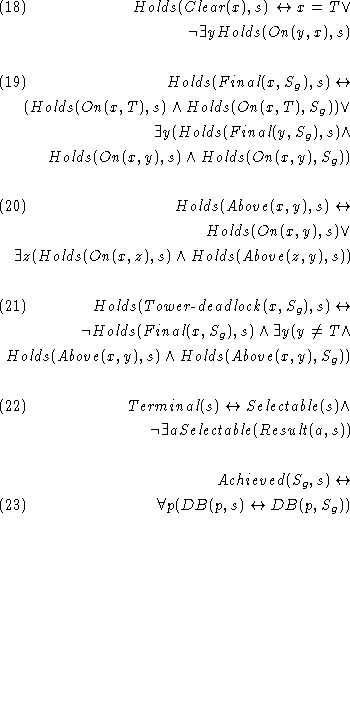. In general, a problem will be described by a set of conditions on some initial and goal situations. The particular problem described by axiomsandcharacterizes completely the state of the initial and goal situations, but this needs not be the case. Specifying a set of constrains on initial and goal situations allows defining classes of problems, instead of particular instances. Such constraints can be used then to reason about the behavior of different strategies on a class of problems.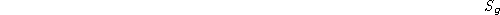The formulas presented so far allow us to prove that some actions are good, bad or better than others for a given goal and a particular situation. But we aren't still able to decide which situations are selectable according to a particular strategy. Action selection rules do not give us complete information. They don't tell us which actions are ``not good'', ``not bad'', or ``not better'' than others. In order to interpret them in terms of action selection (using axiom), we need to be able to jump to the conclusion that an action is ''not good'' or ``not bad'' unless the heuristics known so far (axiomsto) imply that it is so. This incompleteness of our formalization is also one of its main advantages, because it allows us to refine the problem solving strategy of a program by simple additions of better heuristics. We illustrate this idea with an advice taking scenario later on.

The nested abnormality theory described below characterizes the behavior of the strategy described by axiomstowhen it is applied to solve the problem described by axiomsand. That is, it allows us to determine what situations (and, therefore, what sequences of actions) can be selected according to the strategy. Let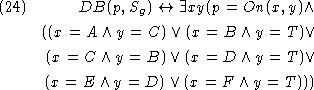be the conjunction of the universal closures of the formulasto.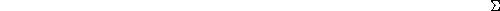The expressiondescribes a nested abnormality theory in which circumscription is applied in the following way. The predicate Good is circumscribed with respect to the universal closures of the axioms describing the strategy of the program (axiomsto). The predicate Bad is circumscribed with respect to the result of the circumscription described above and the universal closure of axiom, which contributes to the definition of Bad with positive instances of Better. We need to let Better vary, because minimizing the extension of Bad may affect (through axiom) the extension of Better. The latter circumscription, which characterizes the extensions of the predicates Good and Bad, is conjoined with, which describes the theory of action assumed for the blocks world (axiomsto), the specific problem reasoned about (axiomsand), and the mechanism for action selection (axiom).Next: Theorem 1 Up: Declarative Formalization of Strategies Previous: Introduction

Josefina Sierra
Tue Jul 21 09:54:27 PDT 1998